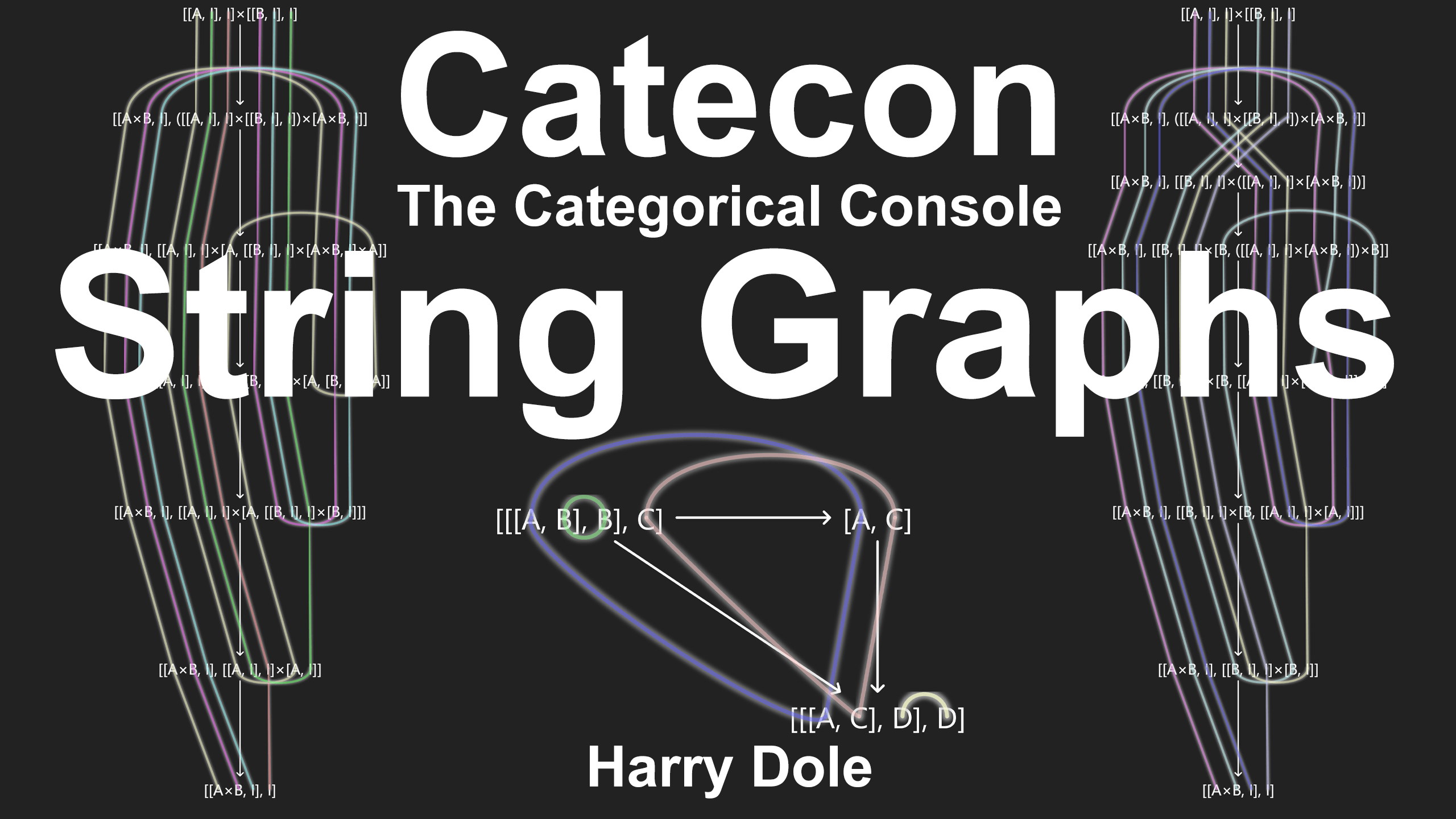# Catecon: String Graphs

See the diagram at https://catecon.net/d/hdole/graph

In this video we show various string graphs in Catecon.

In particular, the origin of the icon for string graphs is given.

Let’s get morphing.

String graphs, or sometimes referred to as Kelly-Mac Lane graphs, see nLab, are used for coherence in closed categories.

In Catecon they visually indicate what some morphisms are doing, and the C++ code generator uses them for determining variables.

Our first examples are just some simple factor morphisms.

Select them and hit the G key or the string graph icon.

Essentially a string tracks the identity in a morphism.

Next we see the two distinct morphisms from A cross A to A cross A, the identity, and the twist.

In Catecon the twist is indicated by a factor morphism where the factors are reversed as indicated by the one followed by zero.

For the product and coproduct, we have the graphs for the delta and fold morphisms.

In Catecon hom objects are indicated by square brackets in the typical manner.

The left side indicates the domain and right side the codomain, and the bracketed expression the space of all such morphisms matching said domain and codomain.

By using the lambda action, or currying, the domains of the delta and fold are moved to the domain side of the codomain’s hom.

These pointed objects then just pick out the morphism out of that space.

When strings touch they should inherit the same color.

Here we see the identity on A cross B versus the product of the identity, and dually.

Distribution has its own interesting form with built-in deltas.

Next up are the identities on homs and products.

Currying those two give us the evaluation map e on the left and the d on the right.

Connections are as expected.

Now curry the hom A to B to the right side of the evaluation to get our next morphism.

Take the hom of that latter morphism with the identity on C.

Note that the left-hand of the hom is what is known as contravariant, meaning the direction of the arrow is apparently reversed as we see here.

Here is another evaluation, and we move the left-hand factor over to the codomain.

We have enough to do some composing, so here we have the result.

Let’s just look at the resulting composite, and there’s the string graph icon with the evident angled element.

Setting B, C, and D to the closed category’s unit yields a morphism from A triple dual to A triple dual that may not be the identity.

Now let’s tackle the Arens multiplications.

These are morphisms from what nominally would be A double dual tensor B double dual to A tensor B double dual.

Now here I’m in the Cat category and not some category of vector spaces, but we’ll make do in the construction using the same means as for classical Arens multiplication.

And here are their graphs.

That’s a bit mysterious so let’s try to get a more detailed look at all the currying that gives us these two morphisms and their very, very long names.

And there we have it.

Let’s take a look at the left side first.

Essentially use the d to add the parameter hom of A cross B to I to the original codomain.

This technique gives us enough to start doing evaluations.

Do it again on the right side by adding A.

In the next stage use the morphism hom A cross B to I to hom A to hom B to I.

By isolating the A we can now form the evaluation to reveal the hom B to I in the next stage.

That is evidently another evaluation, and another to get the final shape.

The right-hand side is similar but has the roles of A and B interchanged.

In the final graph there’s no choice for linking A’s and B’s, so you see the strings get crossed in this setup stage.

In a later stage we uncross when the order of isolation of the variables is chosen.

Quod erat demonstandum.

String graphs in Catecon.